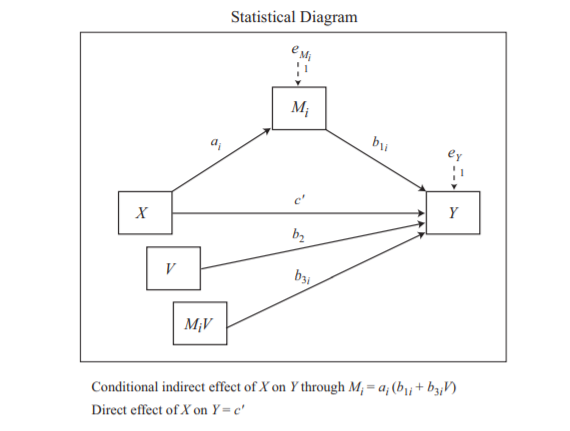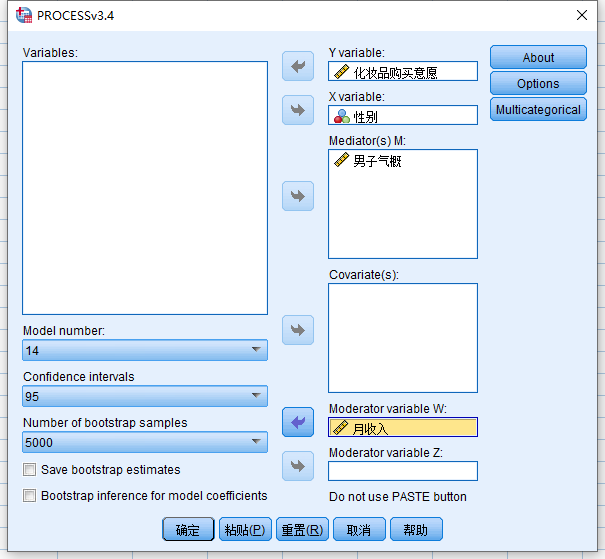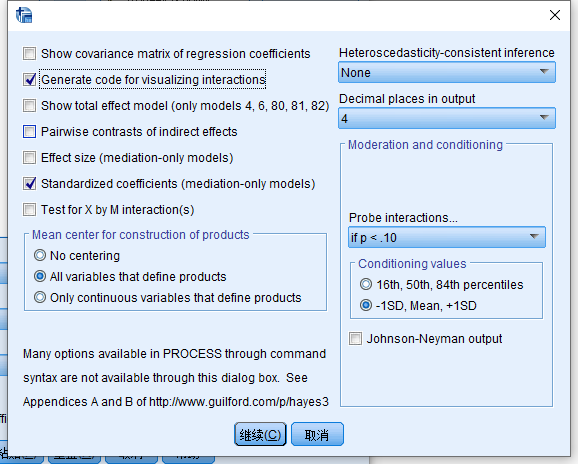• X/M/V/Y分别是自变量/中介变量/调节变量/因变量
• MV变量代表M和V的乘积变量(需要先中心化再求乘积)
• a/b/c都是回归模型中的回归系数

$$(1) M = h_M + aX + e_M$$

$$(2) Y = h_Y + bM + c'X + b_2V + b_3MV + e_Y$$

$$Y = h_Y + bh_m + abX + c'X + b_2V + b_3V(h_M+aX) + e_Y$$

$$ME = ab + ab_3V$$

### SPSS检验过程

• 将M和V进行中心化, 也就是减去各自均值
• 求变量MV
• 以M为被预测变量, X为预测变量, 做回归(检验回归方程1)
• 以Y为被预测变量, X/V/MV/M为预测变量, 做回归(检验回归方程2)
• 使用bootstrap方法求$ab_3$

### 使用Process一键检验

Process没有什么魔力, 只是将spss的五个步骤, 合成到一起, 让你一键得到所有需要的结果。所以我们用一两个图就能看完Process的所有配置:

• 自变量是:性别
• 因变量: 化妆品购买意愿
• 中介变量: 男子气概
• 调节变量: 月收入### 赞助